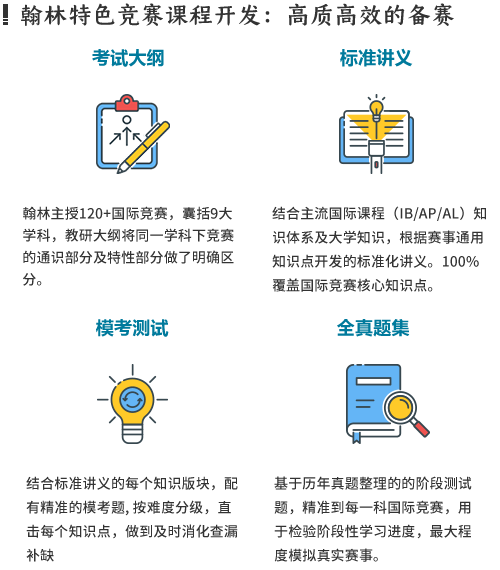# 2021AMC8基础班

1、适合人群：2021年11月8年级以下，无amc8基础，目标为5%的学生

2、AMC8培训班为3-8人小班，满3人开班

## 描述AMC8美国初中数学竞赛由美国数学协会（MAA）组织，每年举办一次，针对八年级及以下学生(对应国内初一初二学生，部分小学四～六年级的优秀学生也可以参加)的数学竞赛，AMC8获得高分的学生在得到学校允许后，将受邀参加AMC10比赛。VIP一对一定制辅导课程

### AMC8课程大纲：

1. Number theory

（1）Even and odd

（2）Prime and composite

（3）Prime factorization

（4）Number of factors

2. Algebra

（1）Positive and negative number

（2）Operations with fractions

（3）Terminating decimals and repeating decimals

（4）Converting repeating decimals to fractions

（5）Operations of irrational numbers

（6）linear equations

（7）Linear inequations and system of linear inequations

（8）Functions

（9）Words problem related to percents

3. Geometry

（1）Lines (line segments, straight lines, radial lines)

（2）Angles (acute angles, obtuse angles, straight angles, round angles, vertical angles, complementary angles, supplementary angles)

（3）Relationship of angles formed by parallel lines

（4）Triangles (acute triangles, obtuse triangles, right triangles, perimeter, area)

4. Pythagorean theorem

（1）Properties of isosceles triangles and equilateral triangles

（2）Probability and Statistic

（3）Statistics (average numbers, mode, median, variance, standard deviation, range, graphs)

（4）Sum rules and product rules

### 部分导师简介

5年美国生活、学习、工作经验。研究生期间担任大学助教，带教的多名学生获得学院奖学金，并有学生顺利拿到名校研究生Offer。

### 咨询请添加顾问老师